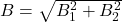Question

. One long wire carries a current of 30 A along the entire x axis. A second-long wire carries a current of 40 A perpendicular to the xy plane and passes through the point (0, 4) m. What is the magnitude of the resulting magnetic field at the point y = 2.0 m on the y axis?

1.magnitude of net magnetic field at given point is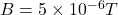Explanation:

As we know that magnetic field due to a long current carrying wire is given as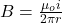here we we will find the magnetic field due to wire which is along x axis is given as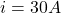r = 2 m

now we have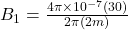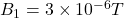into the plane

Now similarly magnetic field due to another wire which is perpendicular to xy plane is given as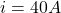r = 2 m

now we have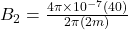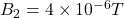along + x direction

Since the two magnetic field is perpendicular to each other

So here net magnetic field is given as